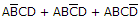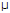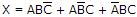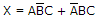# Digital Electronics - Combinational Logic Analysis

### Exercise :: Combinational Logic Analysis - General Questions

11.

To implement the expression, it takes one OR gate and ________.

 A. three AND gates and three inverters B. three AND gates and four inverters C. three AND gates D. one AND gate

Answer: Option A

Explanation:

No answer description available for this question. Let us discuss.

12.

One positive pulse with tw = 75 µs is applied to one of the inputs of an exclusive-OR circuit. A second positive pulse with tw = 15 µs is applied to the other input beginning 20 µs after the leading edge of the first pulse. Which statement describes the output in relation to the inputs?

 A. The exclusive-OR output is a 20s pulse followed by a 40s pulse, with a separation of 15s between the pulses. B. The exclusive-OR output is a 20s pulse followed by a 15s pulse, with a separation of 40s between the pulses. C. The exclusive-OR output is a 15s pulse followed by a 40s pulse. D. *The exclusive-OR output is a 20s pulse followed by a 15s pulse, followed by a 40s pulse.

Answer: Option D

Explanation:

No answer description available for this question. Let us discuss.

13.

How many AND gates are required to implement the Boolean expression,?

 A. 1 B. 2 C. 3 D. 4

Answer: Option C

Explanation:

No answer description available for this question. Let us discuss.

14.

How many NOT gates are required to implement the Boolean expression,?

 A. 1 B. 2 C. 4 D. 5

Answer: Option B

Explanation:

No answer description available for this question. Let us discuss.

15.

The inverter can be produced with how many NAND gates?

 A. 1 B. 2 C. 3 D. 4

Answer: Option A

Explanation:

No answer description available for this question. Let us discuss.

#### Current Affairs 2021

Interview Questions and Answers